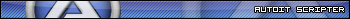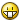# Not working, IsNumber ; StringSplit(\$sFileRead, '=')

## Recommended Posts

```\$splits = StringSplit(\$sFileRead, '=')
If (\$splits > 1) Then
If (IsNumber(\$splits) And \$splits <= 10) Then
ConsoleWrite(\$splits & "::" & \$splits & @CRLF)
EndIf
EndIf```

I'm not sure if its just me or what is going on but its not working how i expected???

I am just cleaning up an ini file.
If the value is greater then 10 then we want to delete that line. Also has to bypass keys or values that are not numbers. I'm also open to more effective approaches to accomplishing the task.  Such as for loop _FileCountLines but that didn't work right for me. so i did if error flag exitloop which works well.

Full code in quotes.

Quote

Full code

```#include <FileConstants.au3>
#include <MsgBoxConstants.au3>
#include <WinAPIFiles.au3>
#include <File.au3>

ConsoleWrite("BOOT" & @CRLF&@CRLF)
Example()

Func Example()
Local Const \$sFilePath = (@ScriptDir & "\data.ini")
; Open the file for reading and store the handle to a variable.
If \$hFileOpen = -1 Then
ConsoleWrite("An error occurred when reading the file." & @CRLF)
Return False
Else
; Read the fist line of the file using the handle returned by FileOpen.
For \$i = 1 To 1000000
If (@error) Then
ExitLoop
Else
If (\$splits > 1) Then
If (IsNumber(\$splits) And \$splits <= 10) Then
ConsoleWrite(\$splits & "::" & \$splits & @CRLF)
EndIf
EndIf
EndIf
Next
EndIf
; Close the handle returned by FileOpen.
FileClose(\$hFileOpen)
EndFunc   ;==>Example```

If the variable is a numeric value represented as a string, then IsNumber() will return 0.

I suspect I would have to chr cycle to verify a value. then push it to a int()?

Edited by major_lee
...
##### Share on other sites

goes to show what a little reading gets you.. but still , maybe there is a better way?

Quote

Working Code

```\$splits = StringSplit(\$sFileRead, '=')
If (\$splits > 1) Then
If (IsNumber(Number(\$splits)) And Number(\$splits) <= 10 And Number(\$splits) <> 0) Then
ConsoleWrite(\$splits & "::" & \$splits & @CRLF)
EndIf
EndIf```

##### Share on other sites

```Func IsComfortablyNumber(\$string) ; reference https://www.youtube.com/watch?v=_FrOQC-zEog
If Number(\$string) == Int(\$string) And Number(\$string) == \$string Then Return SetError(0, 2, Int(\$string)) ; is Integer
If Number(\$string) == \$string Then Return SetError(0, 1, Number(\$string)) ; is Real
Return SetError(0, 0, \$string)
EndFunc```

I have not seen the file you read, so, would this work ?.

Edited by argumentum
better code##### Share on other sites

19 minutes ago, major_lee said:

IsNumber(Number(\$splits))

Do you sometimes get this false ?

BTW what about the Ini* functions ?

##### Share on other sites

1 minute ago, mikell said:

Do you sometimes get this false ?

BTW what about the Ini* functions ?

The problem was,

`IsNumber(\$splits) `
`                    If (IsNumber(\$splits) And \$splits <= 10) Then`

Documentation remarks says, If the variable is a numeric value represented as a string, then IsNumber() will return 0.

Therefor the solution is.

```
If (IsNumber(Number(\$splits)) And Number(\$splits) <= 10 And Number(\$splits) <> 0) Then```
##### Share on other sites

Try this

`msgbox(0,"", IsNumber(Number("test")) )`

and this

```\$var = "11"
msgbox(0,"", \$var > 10 )```

##### Share on other sites

```ConsoleWrite(@ScriptLineNumber & @TAB & IsComfortablyNumber("-1.5") & @TAB & @extended & @CRLF) ; Return @extended = yes/real
ConsoleWrite(@ScriptLineNumber & @TAB & Number("-1.5") & @CRLF)
ConsoleWrite(@ScriptLineNumber & @TAB & IsNumber("-1.5") & @CRLF & @CRLF)

ConsoleWrite(@ScriptLineNumber & @TAB & IsComfortablyNumber("0") & @TAB & @extended & @CRLF) ; Return @extended = yes/integer
ConsoleWrite(@ScriptLineNumber & @TAB & Number("0") & @CRLF)
ConsoleWrite(@ScriptLineNumber & @TAB & IsNumber("0") & @CRLF & @CRLF)

ConsoleWrite(@ScriptLineNumber & @TAB & IsComfortablyNumber("a") & @TAB & @extended & @CRLF) ; Return @extended = no
ConsoleWrite(@ScriptLineNumber & @TAB & Number("a") & @CRLF)
ConsoleWrite(@ScriptLineNumber & @TAB & IsNumber("a") & @CRLF & @CRLF)

If Number(\$string) == Int(\$string) And Number(\$string) == \$string Then Return SetError(0, 2, Int(\$string)) ; is Integer
If Number(\$string) == \$string Then Return SetError(0, 1, Number(\$string)) ; is Real
Return SetError(0, 0, \$string)
EndFunc```

the OP is happy with the solution but, ...I believe that what I presented is more real. I mean, zero is a numberEdited by argumentum
better code##### Share on other sites

Here is everything.  Looks solid now.

summary of data.ini

Quote

[info]
counter=256
name=Dub
[checks]
3508083115=1
3269532009=3
3183286609=33
3402111378=270
3353486253=2
3855754413=5
4094305009=4
3162121333=3
3164218273=5
3494781865=4
3712885941=3
4177404797=4
3537249249=3
3348441145=2
1578968073=2
[zone]
701826551=
761202179=
895682105=
3402111378=

clearData.au3

```#include <FileConstants.au3>
#include <MsgBoxConstants.au3>
#include <WinAPIFiles.au3>
#include <File.au3>

ConsoleWrite("BOOT" & @CRLF&@CRLF)
Example()

Func Example()
Local Const \$sFilePath = (@ScriptDir & "\data.ini")
Local Const \$sFilePath1 = (@ScriptDir & "\temp.ini")
; Open the file for reading and store the handle to a variable.
FileDelete(\$sFilePath1)
Local \$hFileOpen = FileOpen(\$sFilePath, \$FO_READ )
Local \$hFileOpen1 = FileOpen(\$sFilePath1, \$FO_APPEND)
If \$hFileOpen = -1 Or \$hFileOpen1 = -1  Then
ConsoleWrite("An error occurred when reading the file." & @CRLF)
Return False
Else
; Read the fist line of the file using the handle returned by FileOpen.
For \$i = 1 To _FileCountLines ( \$sFilePath )
If (@error) Then
ConsoleWrite(\$i&":"&"ExitLoop" & @CRLF)
ExitLoop
Else
If (\$splits <= 1) Then
Else
If (IsNumber(Number(\$splits)) And Number(\$splits) <= 10 And Number(\$splits) <> 0) Then
ConsoleWrite(\$i&":wipe:"&\$splits & "=" & \$splits & @CRLF)
Else
ConsoleWrite(\$i&":"&\$splits & "=" & \$splits & @CRLF)
EndIf
EndIf
EndIf
Next
EndIf
FileClose(\$hFileOpen)
FileCopy(\$sFilePath1,\$sFilePath, \$FC_OVERWRITE )
EndFunc   ;==>Example```

Edited by major_lee
##### Share on other sites

10 minutes ago, argumentum said:
```ConsoleWrite(@ScriptLineNumber & @TAB & IsComfortablyNumber("1") & @TAB & @extended & @CRLF) ; Return @extended = yes
ConsoleWrite(@ScriptLineNumber & @TAB & Number("1") & @CRLF)
ConsoleWrite(@ScriptLineNumber & @TAB & IsNumber("1") & @CRLF & @CRLF)

ConsoleWrite(@ScriptLineNumber & @TAB & IsComfortablyNumber("0") & @TAB & @extended & @CRLF) ; Return @extended = yes
ConsoleWrite(@ScriptLineNumber & @TAB & Number("0") & @CRLF)
ConsoleWrite(@ScriptLineNumber & @TAB & IsNumber("0") & @CRLF & @CRLF)

ConsoleWrite(@ScriptLineNumber & @TAB & IsComfortablyNumber("a") & @TAB & @extended & @CRLF) ; Return @extended = no
ConsoleWrite(@ScriptLineNumber & @TAB & Number("a") & @CRLF)
ConsoleWrite(@ScriptLineNumber & @TAB & IsNumber("a") & @CRLF & @CRLF)

If Int(\$string) == \$string Then Return SetError(0, 1, Int(\$string))
Return SetError(0, 0, \$string)
EndFunc```

the OP is happy with the solution but, ...I believe that what I presented is more real. I mean, zero is a numberYes, my issue was thinking about a string not knowing its a int. while the return was 0 still being a number.
In this situation i can work around it.
As understood 0 being a number and if the string was 0 in the first place then there would be a conflict.

I think what would happen is

splits = 0

THEN

IsNumber(Number(\$splits))  = 1

##### Share on other sites

```ConsoleWrite(IsNumber(Number("")) & @CRLF) ; = 1
ConsoleWrite(IsNumber(Number("1")) & @CRLF) ; = 1
ConsoleWrite(IsNumber(Number("0")) & @CRLF) ; = 1
ConsoleWrite(IsNumber(Number("a")) & @CRLF & @CRLF) ; = 1```

It all return one```Global \$aArray = ["0", "1", "a", ""]
For \$v = 0 To 3 ; it's only true with the "1", so it does what you need, hence, good  =)
ConsoleWrite(  (IsNumber(Number(\$aArray[\$v])) And Number(\$aArray[\$v]) <= 10 And Number(\$aArray[\$v]) <> 0)  & @CRLF)
Next
Exit```

But you're looking for an int greater than zero, so it's all good== School time ==

Number: Returns the numeric representation of an expression
IsNumber: Checks if a variable's base type is numeric.

So, Number returns a 0 or a 1, both integers. And you ask is IsNumber(), and that is what Number() returns, so, this logic goes nowhere fast.
But then you ask: are you less than ten and not a zero ? and the answer is what you expected.

What @mikell presented:

```Global \$aArray = ["0", "1", "a", ""]
For \$v = 0 To 3 ; it's only true with the "1", so it does what you need, hence, good  =)
;~  ConsoleWrite(  (IsNumber(Number(\$aArray[\$v])) And Number(\$aArray[\$v]) <= 10 And Number(\$aArray[\$v]) <> 0)  & @CRLF)
ConsoleWrite(  ( \$aArray[\$v] > 0 And \$aArray[\$v] <= 10 )  & @CRLF) ; @mikell
Next```

is what you need.##### Share on other sites

4 minutes ago, argumentum said:
```ConsoleWrite(IsNumber(Number("")) & @CRLF) ; = 1
ConsoleWrite(IsNumber(Number("1")) & @CRLF) ; = 1
ConsoleWrite(IsNumber(Number("0")) & @CRLF) ; = 1
ConsoleWrite(IsNumber(Number("a")) & @CRLF & @CRLF) ; = 1```

It all return one```Global \$aArray = ["0", "1", "a", ""]
For \$v = 0 To 3 ; it's only true with the "1", so it does what you need, hence, good  =)
ConsoleWrite(  (IsNumber(Number(\$aArray[\$v])) And Number(\$aArray[\$v]) <= 10 And Number(\$aArray[\$v]) <> 0)  & @CRLF)
Next
Exit```

But you're looking for an int greater than zero, so it's all good== School time ==

Number: Returns the numeric representation of an expression
IsNumber: Checks if a variable's base type is numeric.

So, Number returns a 0 or a 1, both integers. And you ask is IsNumber(), and that is what Number() returns, so, this logic goes nowhere fast.
But then you ask: are you less than ten and not a zero ? and the answer is what you expected.

What @mikell presented:

```Global \$aArray = ["0", "1", "a", ""]
For \$v = 0 To 3 ; it's only true with the "1", so it does what you need, hence, good  =)
;~  ConsoleWrite(  (IsNumber(Number(\$aArray[\$v])) And Number(\$aArray[\$v]) <= 10 And Number(\$aArray[\$v]) <> 0)  & @CRLF)
ConsoleWrite(  ( \$aArray[\$v] > 0 And \$aArray[\$v] <= 10 )  & @CRLF) ; @mikell
Next```

is what you need.

I thought I was going to have to do this.  just a rough draft.

```\$string = "945"
ConsoleWrite(@CRLF & "isStringNumber: " & \$string & " - " & isStringNumber(\$string) &  @CRLF)

\$string = "uys"
ConsoleWrite(@CRLF & "isStringNumber: " & \$string & " - " &  isStringNumber(\$string) &  @CRLF)

Func isStringNumber(\$str)
Local \$iLength = StringLen(\$str)
\$return = False
For \$i = 1 To \$iLength
For \$ii = 48 To 57
If Asc ( StringLeft(\$str, 1) ) == \$ii Then
\$return = True
ExitLoop
Else
\$return = False
EndIf
Next
\$str = StringTrimLeft(\$str, 1)
Next
Return \$return
EndFunc   ;==>isStringNumber```

##### Share on other sites

2 hours ago, argumentum said:
```ConsoleWrite(IsNumber(Number("")) & @CRLF) ; = 1
ConsoleWrite(IsNumber(Number("1")) & @CRLF) ; = 1
ConsoleWrite(IsNumber(Number("0")) & @CRLF) ; = 1
ConsoleWrite(IsNumber(Number("a")) & @CRLF & @CRLF) ; = 1```

It all return oneQuite strange, when checking if a number is a number## Create an account

Register a new account

• ### Recently Browsing   0 members

×

• Wiki

• Back

• #### Beta

• Git
• FAQ
×
• Create New...